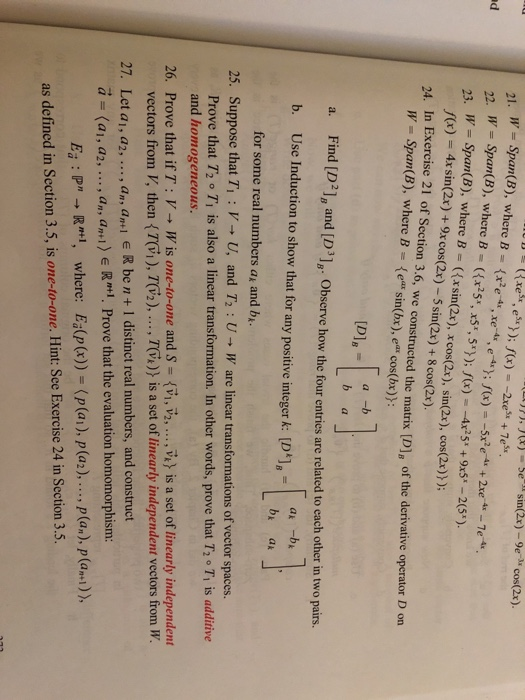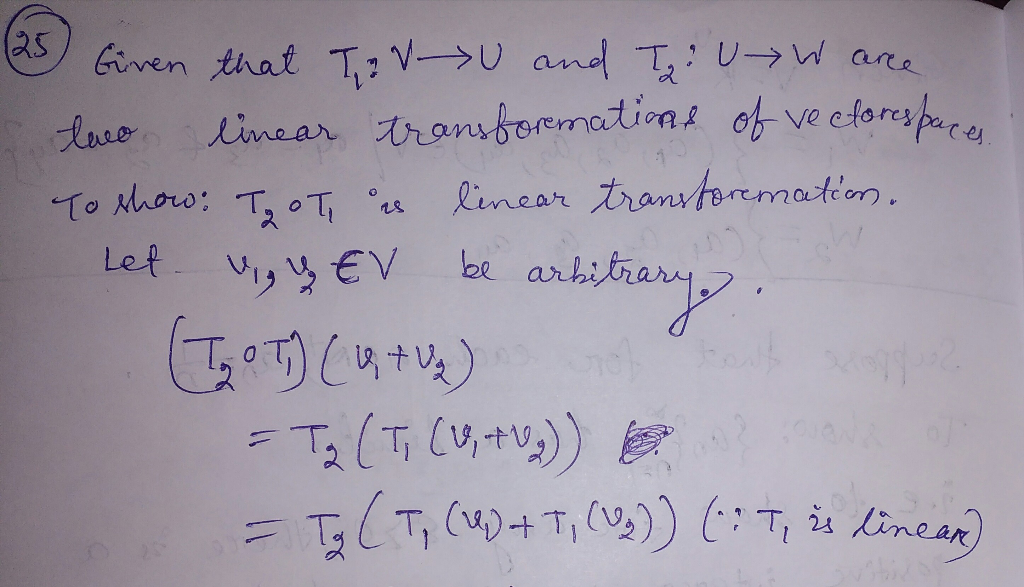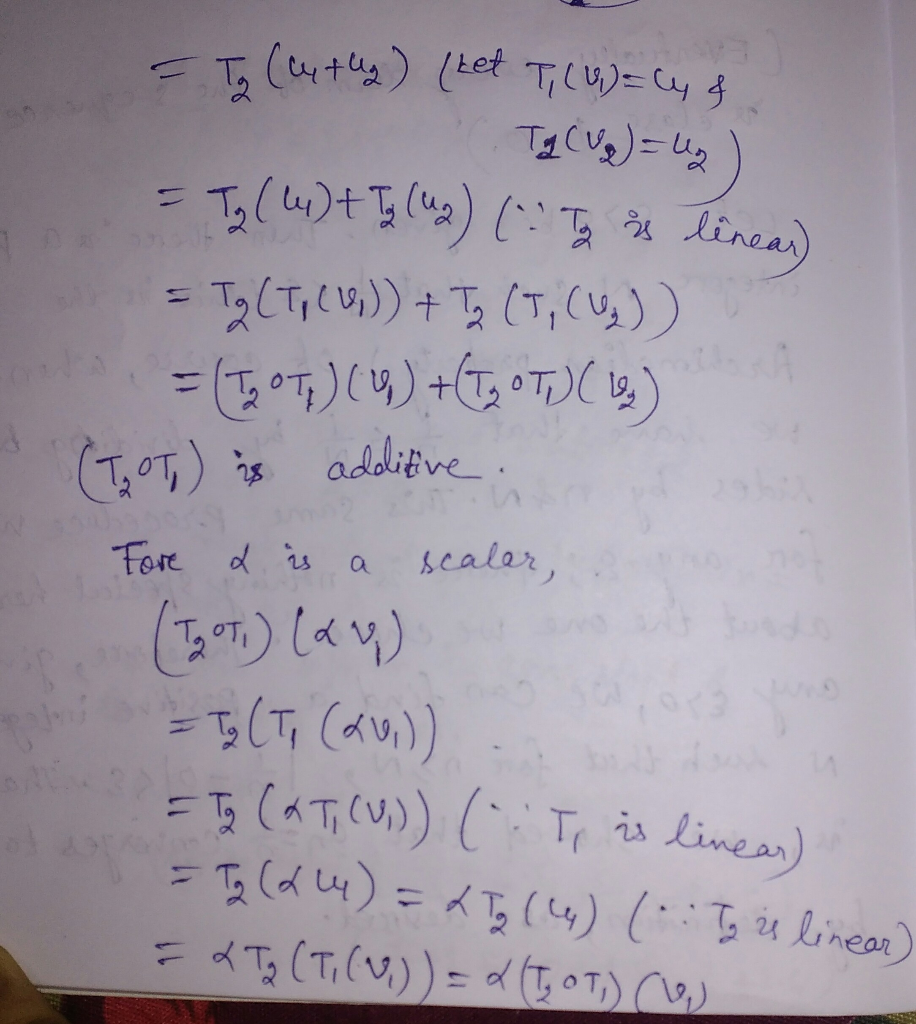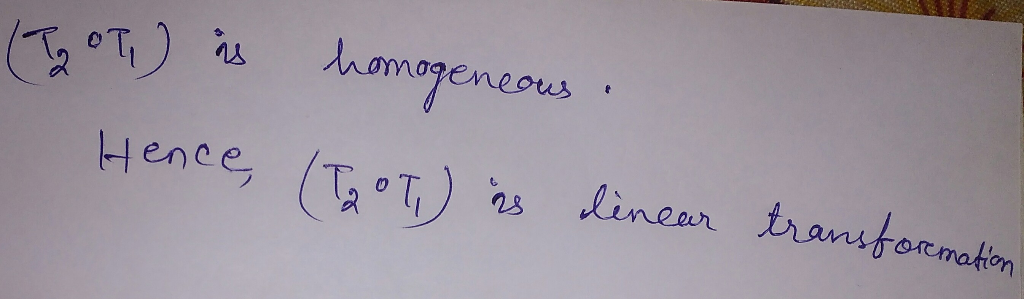Question

# Problem 25 please -Sesin(2x)-9ecos(2x). 21. W = Span(B), where Br(x2e-4x , xe®, e-4x); f(x)--5x2r" + 2e-4-1e...Problem 25 please
-Sesin(2x)-9ecos(2x). 21. W = Span(B), where Br(x2e-4x , xe®, e-4x); f(x)--5x2r" + 2e-4-1e 22. W= Span(B),where B= ({x25, x5*, 5x)); f(x)--4x2 5x+9s5x-2(5x). 3 W Span(B), where B (Exsin(2x), xcos(2x), sin(2x), cos(2x)y): f(x) = 4x sin(2x) + 9x cos(20-5 sin(2x) + 8 cos(2x). 24, In Exercise 21 of Section 3.6, we constructed the matrix [D, of the derivative operator D on W- Span(B), where B e sin(bx), e" cos(bx)): Dls a a. Find [D 1g and [D'lg: Observe how the four entries are related to each other in two pairs. b. Use Induction to show that for any positive integer k: [D, for some real numbers a and bk. 25. Suppose that Ti : V→ U, and T2 : U→ W are linear transformations of vector spaces. Prove that 72。7, is also a linear transformation. In other words, prove that T2。7, is additive and homogeneous. Prove that if T : V → W is one-to-one and S = {vl, V2, vectors from p, then { Twi ),702), , TOİ)} is a set of linearly independent vectors from W , → is a set of linearly independent 26. 27. Let a1, a2,.... an, antl E R ben+ 1 distinct real numbers, and construct Prove that the evaluation homomorphism: a-(ai , аг, . . . , a", a"n) e Rm+1. E : P R, where: Ep(x) (p(a1), p(a).... p la) p(a.)) as defined in Section 3.5, is one-to-one. Hint: See Exercise 24 in Section 3.5.#### Earn Coins

Coins can be redeemed for fabulous gifts.

Similar Homework Help Questions